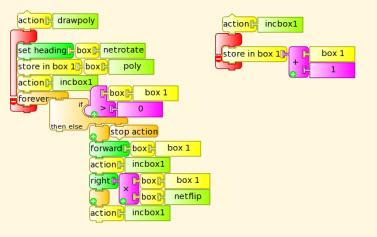Sunday, April 03, 2011

Turtle flip and rotate, 4th grade mathsAn activity in Turtle Art to meet the 4th grade curriculum item "4.G.7 Describe and apply techniques such as reflections (flips), rotations (turns), and translations (slides) for determining if two shapes are congruent"

A number of shapes are randomly generated (in this prototype just a right angle triangle and a trapezium) unrotated and unflipped on the left and randomly rotated and flipped on the right. The student flips and rotates to match the two polygons.In boxes (variables) 11 to 19, the trapezium is stored, distance, rotation, distance, rotation ... and terminated buy -1. Similarly, the triangle is stored in variables 21 to 27.

poly is a random number, either 10 for the trapezium or 20 for the triangle. Rotate is a random number, (0-7) x 45 and flip is either -1 or 1.The program loops, waiting either a 'F' key, 102 or a 'R' key, 114. The polygon is drawn once on the left unflipped and unrotated (netflip =12 and netrotate =0) and drawn once on the right flipped and rotated (netflip = flip x userflip and netrotate = rotate + userrotate)drawpoly draws the polygon specified in box 11-19 or 21-27 terminating on a -1 value, the polygon has rotation and flip specified in netrotate and netflip.

The activity could be improved with a score or reward system, sideways translation, more polygons and randomly congruent and incongruent pairs

Source

Labels: , , , ,